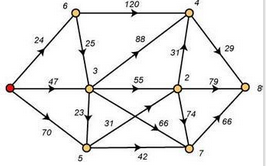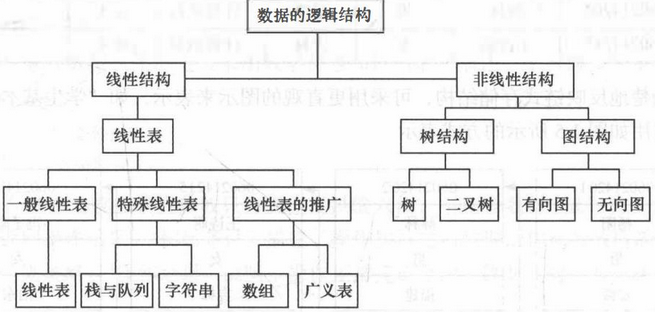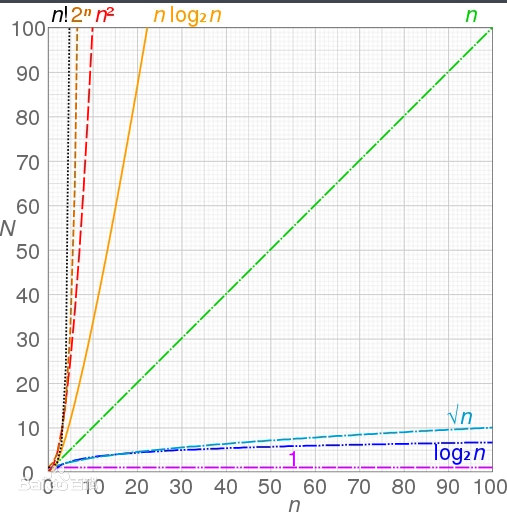WHCSRL 技术网

# 数据结构基础概念与术语等

## 案例导入## 数据结构## 算法时间复杂度和空间复杂度（划重点）

### 时间复杂度#### 常数阶O(1)

``````for(i=0;i<10000;i++){x++;s=0;}
```1```

``````printf("%%d",a);
```1```

#### 线性阶O(n)

``````for(i=0;i<=n;i++){
x++;
}
```123```

#### 平方阶O(n^2)

``````for(i=0;i<=n;i++){
for(j=0;j<=n;j++){
x++;
}
}
```12345```

#### 立方阶O(n^3)

…嵌套三次即可

``````for(i=0;i<=n;i++){
for(j=0;j<=n;j++){
for(k=0;k<=n;k++){
x++;
}
}
}
```1234567```

#### 对数阶O(logn)

``````for(i=1;i<=n;i=2*i){
x++;
}
```123```

x<=logn